# Modules and Packages in Python

## Modules and Packages in Python with Examples

In this article, I am going to discuss Modules and Packages in Python with Examples. Please read our previous article where we discussed Decorators and Generators in Python with examples. As part of this article, we are going to discuss the following pointers which are related to Modules and Packages in Python.

1. What is a module?
2. Renaming or aliasing a module
3. dir() function
4. What is a package?
6. Multiple Programs to understand above concept.
##### What are Modules in Python?

In python a module means a saved python file. This file can contain a group of classes, methods, functions and variables. Every file with .py or .python extension is called a python file, in turn a module.

###### Example: Modules in Python (addmultiplication.py)
```x = 10
def sum(a, b):
print("Sum of two values: " , (a+b))

def multiplication(a, b):
print("Multiplication of two values: " , (a*b))```

Now addmultiplication.py file is a module. addmultiplication.py module contains one variable and two functions.

##### What is import keyword in python?

If we want to use other members(variable, function, etc) of a module in your program, then you should import that module by using the import keyword. After importing you can access members by using the name of that module.

###### Example: demo1.py
```import addmultiplication
###### Output: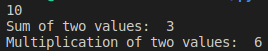Note: Whenever we are using a module in our program, that module’s compiled file will be generated and stored in the hard disk permanently.

##### Renaming or Aliasing a module in Python:

The syntax for renaming or giving an alias name for the module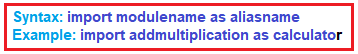###### Example: Aliasing module in python (demo2.py)
```import addmultiplication as calculator
print(calculator.x)
calculator.sum(1, 2)
calculator.multiplication(3, 4)```
###### Output: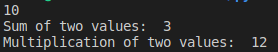##### from keyword in python:

We can import some specific members of the module by using the from keyword. The main advantage of the from keyword is we can access members directly without using module names.

###### Example: from keyword in python (demo3.py)
```from add
multiplication import x, sum
print(x)
sum(10,20)```
###### Output: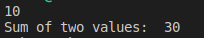##### Import * in python:

We can import all members of a module as by using import * (symbol).

###### Example: import * in python (demo4.py)
```from addmultiplication import *
print(x)
sum(10,20)
multiplication(10,20)```
###### Output: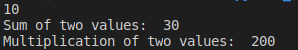##### Aliasing members in Python:

We can give alias name to the members of a module as well

###### Example: Aliasing members of a module in Python (demo5.py)
```from addmultiplication import x as y, sum as add
print(y)
###### Output: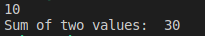Once an alias name is given, we should use the alias name only and not the original name.

###### Example: Aliasing members of a module in Python (demo6.py)
```from addmultiplication import x as y
print(x)```

Output: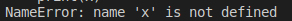By default, a module will be loaded only once even though we are importing multiple times. Let’s consider a module with name module1.

print(“This is from module1”)

Output: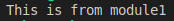```import module1
import module1
import module1
import module1
print("This is test module")```
###### Output: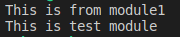The problem in this approach is if a module is updated outside after loading it in our program, then the updated version of the module will not be available to our program. We can solve this problem by reloading modules explicitly based on our requirement wherever needed. We can reload by using the reload() function of the imp module.

Syntax:
import imp

###### Example: demo8.py
```import module1
import module1
print("This is test module")```
###### Output: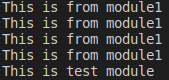In the above program module1 will be loaded 4 times in that 1 time by default and 3 times explicitly. The main advantage of explicit module reloading is we can ensure that updated versions are always available to our program.

##### dir() function in Python:

We can find members of a module by using the dir() function. For every module at the time of execution, the Python interpreter will add some special properties automatically for internal use, for example

__name__
__package__

This inbuilt function dir() helps us to list out all members, including those which are included by interpreter, of the current module or a specified module.

1. dir() ===> To list out all members of current module
2. dir(moduleName) ===> To list out all members of specified module
###### Example: dir() function in python (demo9.py)
```x=10
y=20
def f1():
print("Hello")
print(dir())```
###### Output: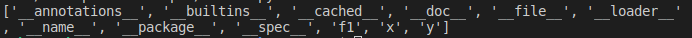###### Example: To display members of the specific module in python (demo10.py)
```import module1
print(dir(module1))
###### Output: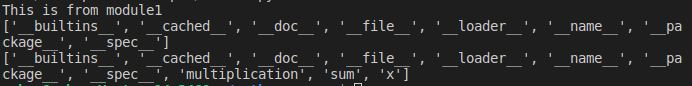##### What is a Package in Python?

A package is nothing but a folder or directory which represents a collection of python modules(programs). Any folder or directory, for python to recognize as a package, should contains__init__ .py file. __init__ .py can be an empty file. A package can contain sub-packages also.

###### Diagrammatic representation of Package in Python: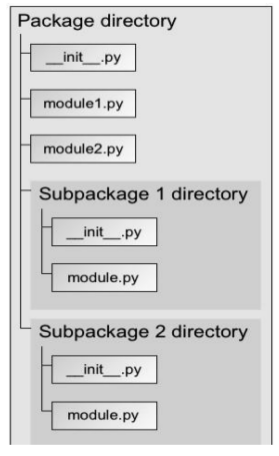##### Summary of Modules and Packages in Python
1. Library → A group of packages
2. Package → A group of modules
3. Modules → A group of functions or methods and classes.